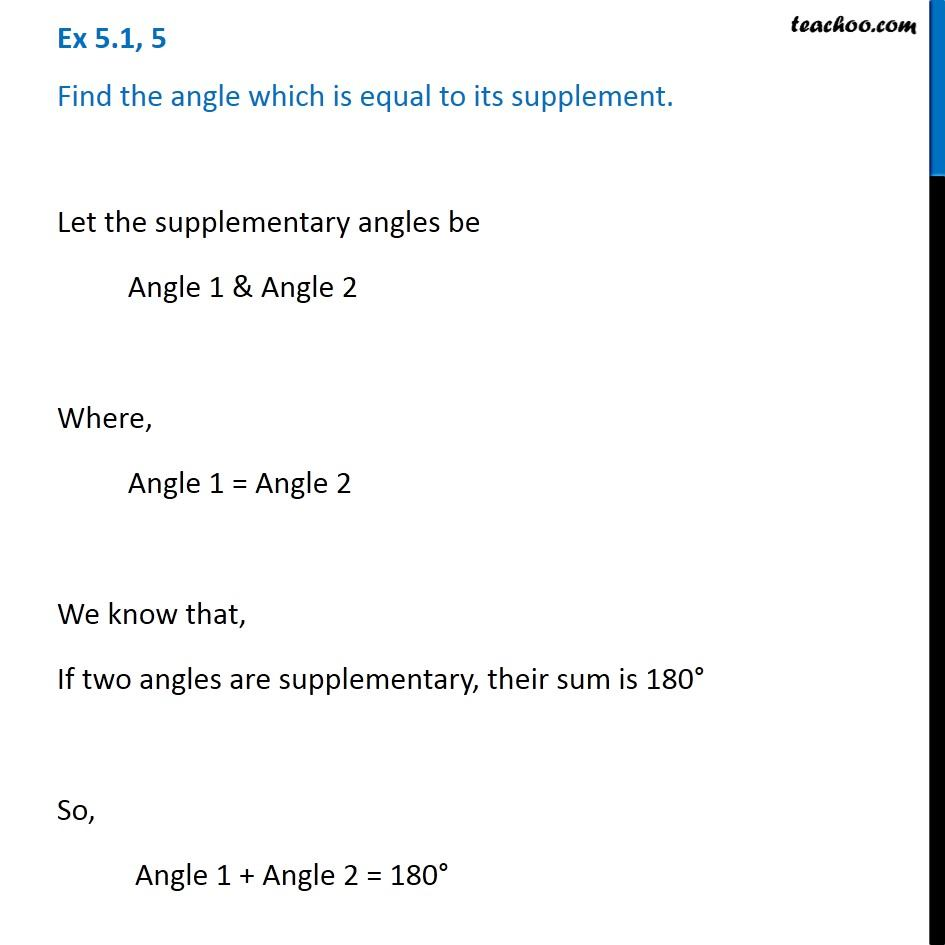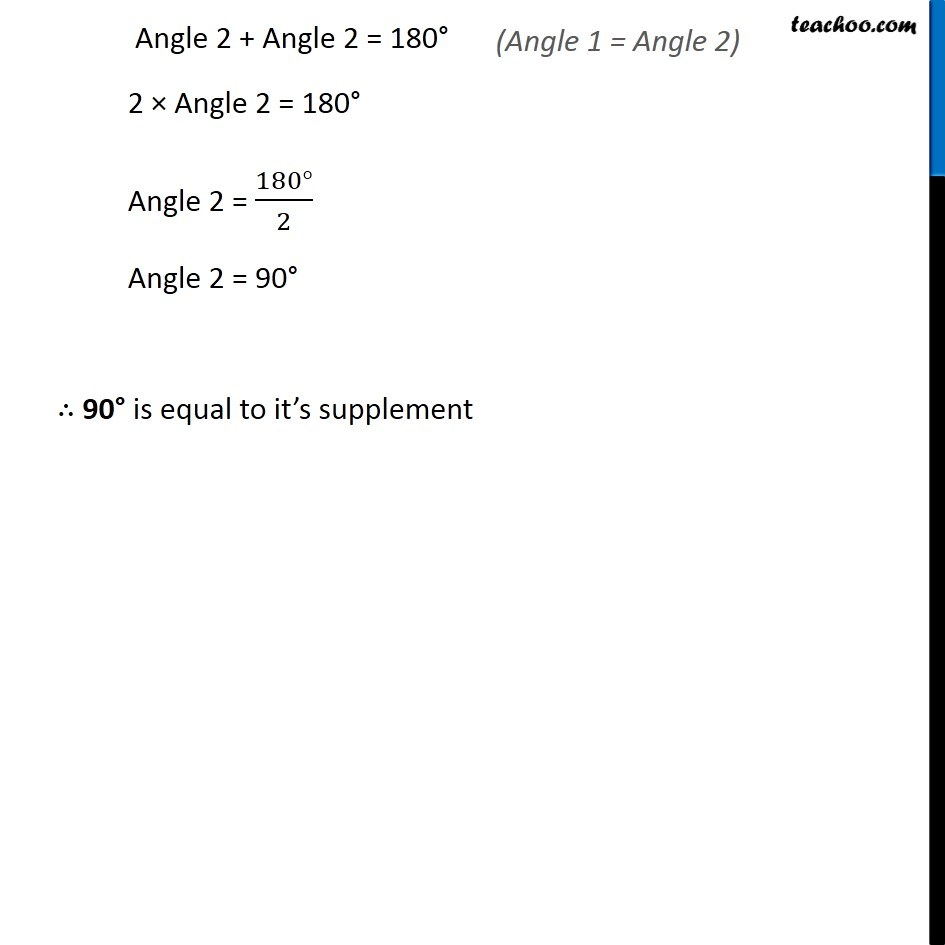1. Chapter 5 Class 7 Lines and Angles
2. Concept wise
3. Supplementary and complementary angles

Transcript

Ex 5.1, 5 Find the angle which is equal to its supplement. Let the supplementary angles be Angle 1 & Angle 2 Where, Angle 1 = Angle 2 We know that, If two angles are supplementary, their sum is 180° So, Angle 1 + Angle 2 = 180° Angle 2 + Angle 2 = 180° 2 × Angle 2 = 180° Angle 2 = (180°)/2 Angle 2 = 90° ∴ 90° is equal to it’s supplement

Supplementary and complementary angles